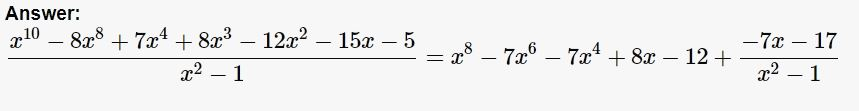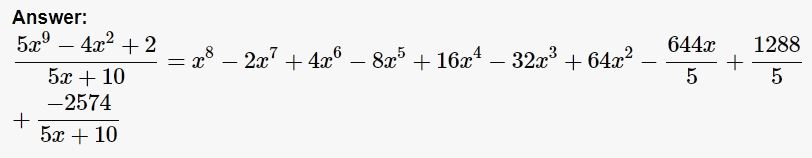# tuffla2022

 यूजर का नाम tuffla2022 स्कोर 113 Membership Statsसवाल 0 जवाब 48

#1
+1

On ex. 1 python says:

There are 33 integers in that group, they are:
15, 45, 75, 105, 135, 165, 195, 225, 255, 285, 315, 345,
375, 405, 435, 465, 495, 525, 555, 585, 615, 645, 675,
705, 735, 765, 795, 825, 855, 885, 915, 945, 975

On ex. 2:

Turning to mod,

in python (and other programming languages)

% is used as the modulus operator

ab%20 = 17

(a + 10) x (b + 10)%20 =

(ab +10a + 10b + 100)%20 =

ab%20 + 10a%20 + 10b%20 + 100%20 =

17 + 10 + 10 + 0 = 17 + 20 = 37 (assuming a and b not divisible by 20)

But 37%20 = 17

6 Agt 2022
#1
+1

As before, use the onilne calculator, here is the answer:

And an image of the solution:The fractional expression is the last term in this, so the remainder is -7x -17.

6 Agt 2022
#1
+1

You can use an online calculator for that, the solution, and the calculator can be found at:

Under is screengrab of the answer:The answer to Your puzzle is thus:

$$-\frac{644x}{5} + \frac{1288}{5} - \frac{2574}{5x + 10}$$

It really is a tedious task to go through all of that.

6 Agt 2022